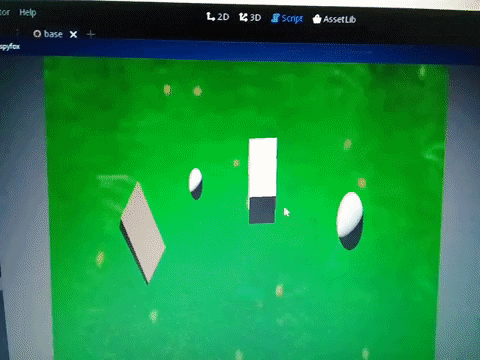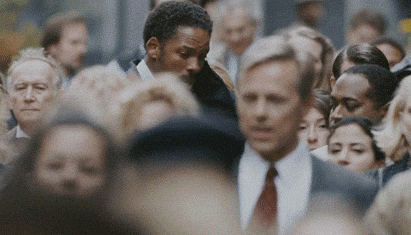i've created a vector, rotated the vector and used to walk, but i do not know how to rotate player to where vector is pointing to
I've tried something like: player.look_at(moveto, Vector3(0,1,0)) but did not work
Thanks

``````extends Spatial
var vetormov= Vector3(0.5,0,0.5)

func _process(delta):

var moveto
if(Input.is_key_pressed(KEY_RIGHT)): angulo = angulo -2
if(Input.is_key_pressed(KEY_LEFT)): angulo = angulo +2

# rotate vector--------------------

# move player using vector---------
if(Input.is_key_pressed(KEY_UP)):
var t = player.get_transform()
t.origin += moveto /10
player.set_transform(t)
``````
in Engine

Why you don't just apply the rotation to your player transform? Transform also has a `rotated` function.

by (1,049 points)

I've tried:
player.global_transform.basis.z= moveto

here player is just a box, when it rotates it get deformed in a certain angle

also tried:

``````t_rot = t.rotated(Vector3(0,1,0),deg2rad(angulo))
player.set_transform(t)
player.set_transform(t_rot)
``````

Still not working

I don't understand why you have two transforms? The basis of a transform is to set all the transform once in an unified manner.

So what's not working? you mean your rotation is not correct? There is no reason that the function rotated is not working as expected.

the mesh is deforming in rotation``````if(Input.is_key_pressed(KEY_UP)):
var t = player.get_transform()
t.origin += moveto /10
t.basis.z= moveto.normalized()
player.set_transform(t)
``````

Thanks this is clearer like that. You can't modify a Basis like that. A Basis is matrix which combine the rotation and scaling, so what you see is normal: read

As the Godot doc already contains useful information, I invite you to read this.

thanks, i've got it, since you've told me the Basis is not the way i've concentrated my efforts in create a vector ahead and "look" at thisvar moveto = Vector3(0,1,0)

``````if(Input.is_key_pressed(KEY_RIGHT)):angulo = angulo -2
if(Input.is_key_pressed(KEY_LEFT)): angulo = angulo +2

#rotate vector---------------------------------------